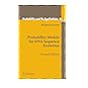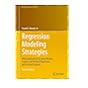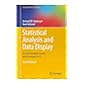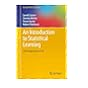Normal view MARC view ISBD view

# Regression Modeling Strategies With Applications to Linear Models, Logistic and Ordinal Regression, and Survival Analysis / by Frank E. Harrell , Jr.

Material type:TextSeries: Springer Series in Statistics: Publisher: Cham : Springer International Publishing : Imprint: Springer, 2015Edition: 2nd ed. 2015.Description: xxv, 582 pages; E 69.99 ill.; 26 cms.Content type: text Media type: computer Carrier type: online resourceISBN: 9783319194240 (hbk.).Additional physical formats: Printed edition:: No titleDDC classification: 519.5 Online resources: Click here to access online
Contents:
Introduction -- General Aspects of Fitting Regression Models -- Missing Data -- Multivariable Modeling Strategies -- Describing, Resampling, Validating and Simplifying the Model -- R Software -- Modeling Longitudinal Responses using Generalized Least Squares -- Case Study in Data Reduction -- Overview of Maximum Likelihood Estimation -- Binary Logistic Regression -- Binary Logistic Regression Case Study 1 -- Logistic Model Case Study 2: Survival of Titanic Passengers -- Ordinal Logistic Regression -- Case Study in Ordinal Regression, Data Reduction and Penalization.- Regression Models for Continuous Y and Case Study in Ordinal Regression -- Transform-Both-Sides Regression -- Introduction to Survival Analysis -- Parametric Survival Models -- Case Study in Parametric Survival Modeling and Model Approximation -- Cox Proportional Hazards Regression Model -- Case Study in Cox Regression -- Appendix.   .
Summary: This highly anticipated second edition features new chapters and sections, 225 new references, and comprehensive R software. In keeping with the previous edition, this book is about the art and science of data analysis and predictive modeling, which entails choosing and using multiple tools. Instead of presenting isolated techniques, this text emphasizes problem solving strategies that address the many issues arising when developing multivariable models using real data and not standard textbook examples. It includes imputation methods for dealing with missing data effectively, methods for fitting nonlinear relationships and for making the estimation of transformations a formal part of the modeling process, methods for dealing with "too many variables to analyze and not enough observations," and powerful model validation techniques based on the bootstrap.  The reader will gain a keen understanding of predictive accuracy, and the harm of categorizing continuous predictors or outcomes.  This text realistically deals with model uncertainty, and its effects on inference, to achieve "safe data mining." It also presents many graphical methods for communicating complex regression models to non-statisticians. Regression Modeling Strategies presents full-scale case studies of non-trivial datasets instead of over-simplified illustrations of each method. These case studies use freely available R functions that make the multiple imputation, model building, validation, and interpretation tasks described in the book relatively easy to do. Most of the methods in this text apply to all regression models, but special emphasis is given to multiple regression using generalized least squares for longitudinal data, the binary logistic model, models for ordinal responses, parametric survival regression models, and the Cox semiparametric survival model.  A new emphasis is given to the robust analysis of continuous dependent variables using ordinal regression. As in the first edition, this text is intended for Masters' or Ph.D. level graduate students who have had a general introductory probability and statistics course and who are well versed in ordinary multiple regression and intermediate algebra. The book will also serve as a reference for data analysts and statistical methodologists, as it contains an up-to-date survey and bibliography of modern statistical modeling techniques. Examples used in the text mostly come from biomedical research, but the methods are applicable anywhere predictive models ("analytics") are useful, including economics, epidemiology, sociology, psychology, engineering, and marketing.
List(s) this item appears in: 2016-10-24
Item type Current location Call number Status Date due Barcode Item holds
Book Chennai Mathematical Institute
General Stacks
519.5 HAR (Browse shelf) Available 9974
Total holds: 0
##### Browsing Chennai Mathematical Institute Shelves , Shelving location: General Stacks Close shelf browser519.5 CRA Mathematical methods of statistics / 519.5 DUR Probability models for DNA sequence evolution / 519.5 GHO Glimpses of India's statistical heritage / 519.5 HAR Regression Modeling Strategies With Applications to Linear Models, Logistic and Ordinal Regression, and Survival Analysis / 519.5 HEI Statistical analysis and data display : an intermediate course with examples in R / 519.5 JAM An introduction to statistical learning : with applications in R / 519.5 OLI Introduction to insurance mathematics : technical and financial features of risk transfers /

Introduction -- General Aspects of Fitting Regression Models -- Missing Data -- Multivariable Modeling Strategies -- Describing, Resampling, Validating and Simplifying the Model -- R Software -- Modeling Longitudinal Responses using Generalized Least Squares -- Case Study in Data Reduction -- Overview of Maximum Likelihood Estimation -- Binary Logistic Regression -- Binary Logistic Regression Case Study 1 -- Logistic Model Case Study 2: Survival of Titanic Passengers -- Ordinal Logistic Regression -- Case Study in Ordinal Regression, Data Reduction and Penalization.- Regression Models for Continuous Y and Case Study in Ordinal Regression -- Transform-Both-Sides Regression -- Introduction to Survival Analysis -- Parametric Survival Models -- Case Study in Parametric Survival Modeling and Model Approximation -- Cox Proportional Hazards Regression Model -- Case Study in Cox Regression -- Appendix.   .